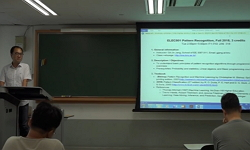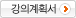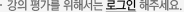### 주메뉴

### 형태인식론

• 경북대학교
• 장길진• 주제분류
공학 >전기ㆍ전자 >전자공학
• 강의학기
2018년 2학기
• 조회수
3,230
•
강의계획서This lecture will cover the basic concepts and principles of pattern recognition and introduce its various applications to help student understand what the pattern recognition is and how it can be used for their research.

#### 차시별 강의1.Lecture 1: Introduction to Pattern Recognition Introduction to Pattern RecognitionLecture 1: Introduction to Pattern Recognition Introduction to Pattern Recognition2.Lecture 2: Probability Theory and Probabilistic Decision Theory Basic Probability TheoryLecture 2: Probability Theory and Probabilistic Decision Theory Basic Probability Theory3.Lecture 3: Bayesian Decision Theory Bayesian Inference and Decision TheoryLecture 3: Bayesian Decision Theory Bayesian Inference and Decision Theory4.Lecture 4: Clustering and K-means Clustering Clustering Vector Quantization (VQ) Pattern Recognition using VQLecture 4: Clustering and K-means Clustering Clustering Vector Quantization (VQ) Pattern Recognition using VQLecture 4: Clustering and K-means Clustering Clustering Vector Quantization (VQ) Pattern Recognition using VQ5.Lecture 5: Normal Random Variable and Its Discriminant Function Designs Normal DistributionsLecture 5: Normal Random Variable and Its Discriminant Function Designs Normal DistributionsLecture 5: Normal Random Variable and Its Discriminant Function Designs Normal Distributions6.Lecture 6: Gaussian Mixture Models and Cross Validation Gaussian Mixture Models (GMM)Lecture 6: Gaussian Mixture Models and Cross Validation Gaussian Mixture Models (GMM)Lecture 6: Gaussian Mixture Models and Cross Validation Gaussian Mixture Models (GMM)7.Lecture 7: Support Vector Machines Support Vector Machines (SVM)Lecture 7: Support Vector Machines Support Vector Machines (SVM)8.Lecture 8: Principal Component Analysis Principal Component AnalysisLecture 8: Principal Component Analysis Principal Component Analysis9.Lecture 9: Single-Layer Linear Perceptron and Multi-Layer Perceptron Single-Layer Linear Perceptron and Multi-Layer PerceptronLecture 9: Single-Layer Linear Perceptron and Multi-Layer Perceptron Single-Layer Linear Perceptron and Multi-Layer Perceptron10.Lecture 10: Handwritten Digit(MNIST) Recognition Using Deep Neural Networks 1 Handwritten Digit(MNIST) Recognition Using Deep Neural Networks 1Lecture 10: Handwritten Digit(MNIST) Recognition Using Deep Neural Networks 1 Handwritten Digit(MNIST) Recognition Using Deep Neural Networks 1Lecture 10: Handwritten Digit(MNIST) Recognition Using Deep Neural Networks 2 Handwritten Digit(MNIST) Recognition Using Deep Neural Networks 2Lecture 10: Handwritten Digit(MNIST) Recognition Using Deep Neural Networks 2 Handwritten Digit(MNIST) Recognition Using Deep Neural Networks 2#### 연관 자료#### 사용자 의견#### 이용방법

• 문서 자료 이용시 필요한 프로그램 [바로가기]

※ 강의별로 교수님의 사정에 따라 전체 차시 중 일부 차시만 공개되는 경우가 있으니 양해 부탁드립니다.